Pressure the Universe | OMICS International
Journal of Physical Mathematics

# Pressure the Universe

Dizhechko BS*

Independent Research, Russia.

*Corresponding Author:
Dizhechko BS
Independent Research
Russia
Tel: 141891141
E-mail: [email protected]

Received Date: June 15, 2016; Accepted Date: July 20, 2016; Published Date: July 27, 2016

Citation: Dizhechko BS (2016) Pressure the Universe. J Phys Math 7:185. doi: 10.4172/2090-0902.1000185

Copyright: © 2016 Dizhechko BS. This is an open-access article distributed under the terms of the Creative Commons Attribution License, which permits unrestricted use, distribution, and reproduction in any medium, provided the original author and source are credited.

Visit for more related articles at Journal of Physical Mathematics

#### Short Commentary

In physics, mass–energy equivalence is a concept formulated by Albert Einstein that explains the relationship between mass and energy. It states every mass has an energy equivalent and vice versa.

The recognition of the concept of mass–energy equivalence requires the recognition of the existence of force of pressure of the universe .

Pressure of the universe is the cause of all the movements taking place in the real world. The greatest it proves itself within the corpuscle, where under his influence shaped portion of energy that defines its mass. Let's deduce concept Universe pressure from the formula of mass-energy equivalence.

E=mc2

It is obvious, that this formula expresses the potential energy passing in kinetic energy when its liberation. Thus the full energy consisting of the sum kinetic W and is equal to potential energy U.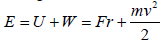Thus, the potential energy enclosed in mass of rest is equal to kinetic energy when it’s full liberation.

Umo=Wmax

In new Cartesian physics corpuscle represents a stationary whirlwind in which he achieved the speed of light, i.e.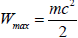and the necessary energy accumulates as a result of compression space on the Compton wavelength, i.e. Umo = Fr , where Compton wavelength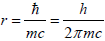. Therefore, we have,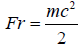From here the force resulting compression of space,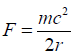Multiply numerator and a denominator of this expression by Compton wavelength, we shall receive,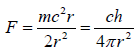Having transformed this expression, we shall receive

F* 4πr2=ch

At the left there is a stream of force through the closed surface, and on the right there is a constant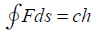Thus, pressure of the Universe is expressed in the form of the Law of a constancy of a stream of forces through the closed surface .

From this we can formulate a law that part of space attracted to each other with a force inversely proportional to the square of the distance between them: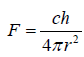In corpuscle, this force is compensated by the centrifugal force of the rotating space reaching the speed of light at a distance of Compton wavelength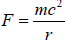from this we get equality.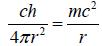After the reduction will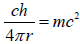.

On the left there is potential energy, the resulting impact on the pressure corpuscle of the universe, and right twice the kinetic energy, i.e. obtain mass-energy equivalence formula.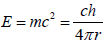Where,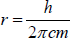Compton wavelength.

Thus, the recognition of the concept of mass–energy equivalence requires the recognition of the existence of force of pressure of the universe and from the formula mass-energy equivalence the formula pressure of the universe follows and, conversely, from the formula of the universe pressure the formula mass-energy equivalence follows.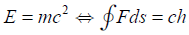Right here is the formula of the law of constancy of flow of forces through a closed surface, which presses on the corpuscle. To the left stands the formula of the law of mass-energy equivalence corpuscles, which counteracts the external flow of forces.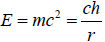From fundamentality of the law of mass-energy equivalence the uniqueness and versatility of the pressure of the universe follows, expressed as the law of constancy of flow of forces through a closed surface shown in Figure 1.

It is necessary to notice, that at formulas of the law of a constancy flux of a vector of forces through the closed surface around of a corpuscle connected with the concept of equivalence of mass and energy there is of Compton wavelength, characterizes compression (stretching) of physical space.

Pressure of the Universe influences on surface corpuscles from all sides so, that the multiplication of force on the area between surfaces of the closed spheres around corpuscle was constant and equal to ch as shown in Figure 2.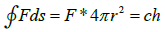Thus, this force is inversely square to the distance to the corpuscles.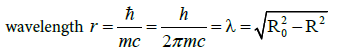In the center of a corpuscle speed of movement of space is close to speed of light and consequently R2 ≈ 0, from here λ ≈ Ro and consequently in a center of a corpuscle formed the kernel in diameter of wave Compton.

To find force of pressure upon a surface of corpuscle ? (?/?2), i.e. how much force presses per unit area, it is obviously necessary to divide this force again on the area of the closed sphere, and we shall receive.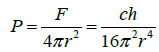If a corpuscle is resting, it would mean that the impact forces of the universe on her from all sides are balanced with each other. If from any party of a corpuscle force of influence of the Universe will decrease in comparison with the opposite party it will move aside smaller influence [3,4].

When the approximation of corpuscles, in an interval between them the universe pressure is reduced due to the fact that the force vectors point in opposite directions. Therefore, corpuscles approach under pressure of the universe from the outside before occurrence the forces of repulsion between them Figure 3. Thus corpuscles stick together with each other.

#### References

Select your language of interest to view the total content in your interested language

### Article Usage

• Total views: 9199
• [From(publication date):
September-2016 - Feb 19, 2020]
• Breakdown by view type
• HTML page views : 9012Can't read the image? click here to refresh